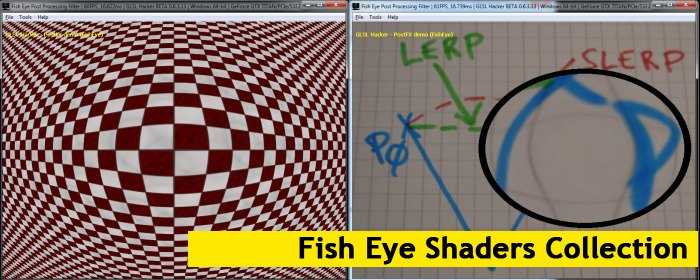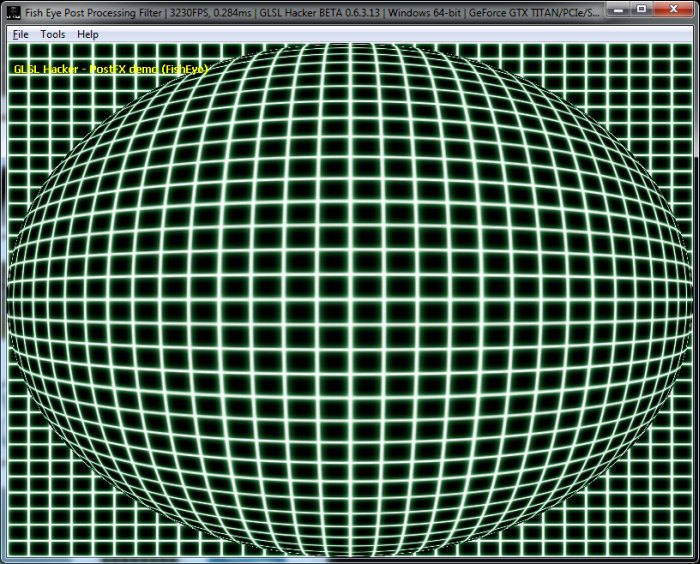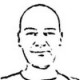(Shader Library) Fish Eye, Dome and Barrel Distortion GLSL Post Processing FiltersArticle index

Here is a collection of some post processing filters in GLSL I found on the web about fish eye and other barrel / dome distortion. For each filter, I coded a small demo with GLSL Hacker that shows the effect in action. You will find all demos in the code sample pack in the host_api/PostFX/FishEye/ folder. All demos require the latest DEV version (0.6.3.13) of GLSL Hacker (Windows, OS X and Linux).

As usual, all the following GLSL shaders can be used in any OpenGL application with minor changes only (shader inputs).

Other types of post processing filters can be found in the Shader Library.#version 120
varying vec4 Vertex_UV;
uniform mat4 gxl3d_ModelViewProjectionMatrix;
void main()
{
gl_Position = gxl3d_ModelViewProjectionMatrix * gl_Vertex;
Vertex_UV = gl_MultiTexCoord0;
}

version 120
uniform sampler2D tex0;
varying vec4 Vertex_UV;
const float PI = 3.1415926535;

void main()
{
float aperture = 178.0;
float apertureHalf = 0.5 * aperture * (PI / 180.0);
float maxFactor = sin(apertureHalf);

vec2 uv;
vec2 xy = 2.0 * Vertex_UV.xy - 1.0;
float d = length(xy);
if (d < (2.0-maxFactor))
{
d = length(xy * maxFactor);
float z = sqrt(1.0 - d * d);
float r = atan(d, z) / PI;
float phi = atan(xy.y, xy.x);

uv.x = r * cos(phi) + 0.5;
uv.y = r * sin(phi) + 0.5;
}
else
{
uv = Vertex_UV.xy;
}
vec4 c = texture2D(tex0, uv);
gl_FragColor = c;
}

Article index

2 thoughts on “(Shader Library) Fish Eye, Dome and Barrel Distortion GLSL Post Processing Filters”

1.fenbf